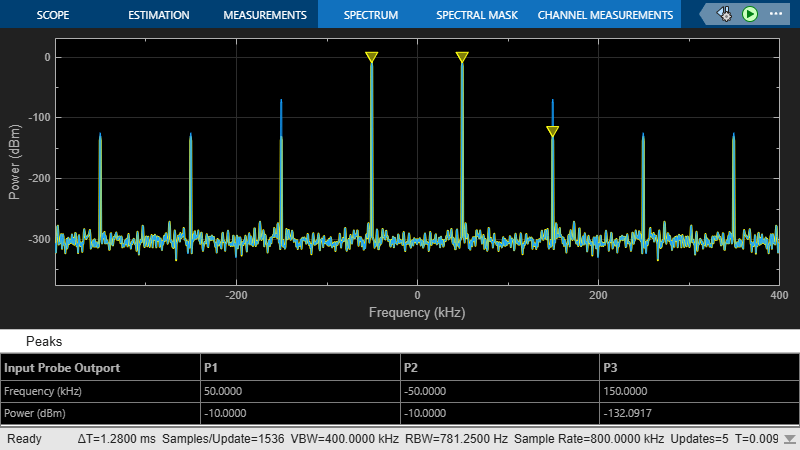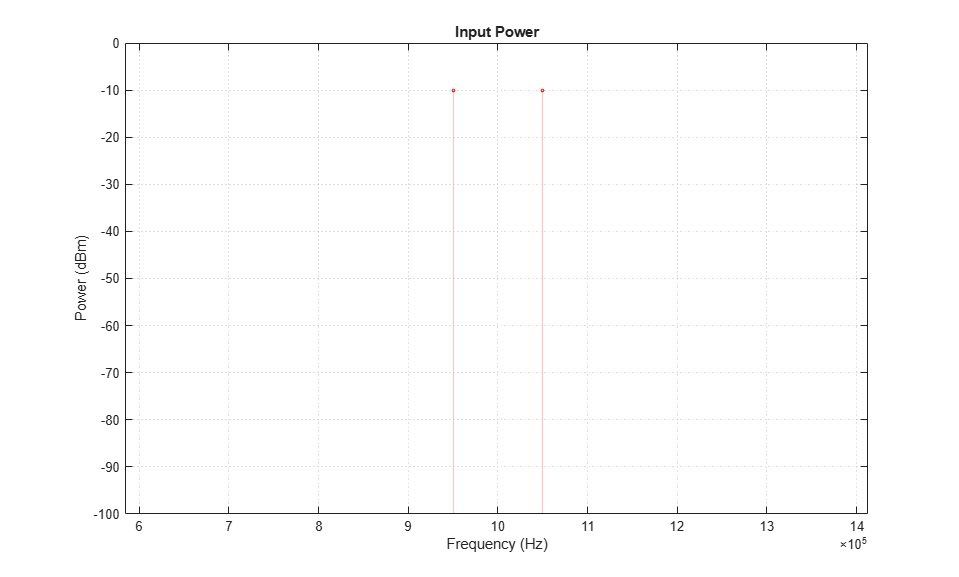Documentation

## Two-Tone Envelope Analysis Using Real Signals

This example shows how to use the RF Blockset™ Circuit Envelope library to test intermodulation distortion of an amplifier using two-carrier envelope analysis.### System Architecture

The system consists of:

• A Simulink® sinusoidal input with frequency `(f1-f2)/2`, and an Inport that assigns the input modulation to the carrier `(f1+f2)/2`. This formulation is equivalent to the sum of two sinusoids with frequency `f1` and `f2`, according to the sum-product formula for cosines:

``
• An amplifier with specified 0 dB linear gain and -20 dB OIP3. For an input signal, the amplifier computes the outputaccording to the polynomial

``
• Two Outport blocks that probe the input and output voltages (across shunt resistors) at the carrier frequency.

• A spectrum analyzer

The example model defines variables for block parameters using a callback function. To access model callbacks, select File > Model Properties > Model Properties and click the Callbacks tab in the Model Properties window.

### Running the Example

1. Type `open_system('simrfV2_power_imd')` at the Command Window prompt.

2. Select Simulation > Run.The resulting output power spectrum, labeled 'Output Power', shows third-order intermodulation distortion. The Display block shows the power of the bandpass waveform, which is half of power of the envelope waveform.

The spectrum analyzer is set to probe for the Output Power. On comparing values, you will see that the spectrum analyzer matches the output power spectrum.

The input drives the nonlinear amplifier into compression, so the linear component of the output is attenuated. To simulate the amplifier in a linear region:

• Specify `Inf` for the IP3 parameter of the Amplifier block located in the Nonlinearity tab of the dialog, or

• Reduce the power of the input signal by decreasing the value of the Amplitude parameter of the Sine Wave block.

### Performing Two-Tone Analysis Using Circuit Envelope Simulation

This example takes advantage of the properties of real signals--namely, the sum-product equivalence of sinusoids. To perform the same experiment on a different RF system:

1. Choose `f1` and `f2`, the frequencies of the test tones.

2. Use a Simulink Sine source and an Inport block to model modulation with frequency `(f1-f2)/2` on a carrier with frequency `(f1+f2)/2`. Alternatively, you can use a Sinusoid block from the RF Blockset Circuit Envelope library to specify both a modulation and a carrier simultaneously.

3. Specify `(f1+f2)/2` as one of your carrier frequencies in the Configuration block dialog.

4. Use an Outport block to probe the distorted signal.

### References

Cripps, Steve C. RF Power Amplifiers for Wireless Communications. Artech House, Inc., 2006.

#### Model RF Power Amplifiers and Increase Transmitter Linearity with DPD Using MATLAB

Download white paper